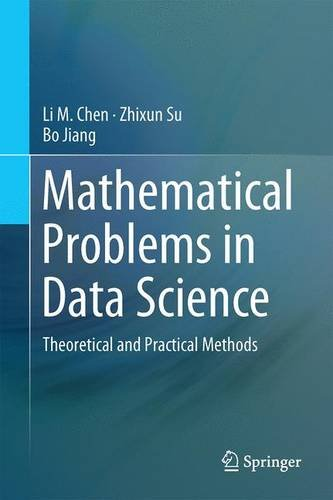# Mathematical Problems in Data Science: Theoretical and Practical Methods

• Length: 213 pages
• Edition: 1st ed. 2015
• Publisher:
• Publication Date: 2016-01-30
• ISBN-10: 3319251252
• ISBN-13: 9783319251257
Description

This book describes current problems in data science and Big Data. Key topics are data classification, Graph Cut, the Laplacian Matrix, Google Page Rank, efficient algorithms, hardness of problems, different types of big data, geometric data structures, topological data processing, and various learning methods.  For unsolved problems such as incomplete data relation and reconstruction, the book includes possible solutions and both statistical and computational methods for data analysis. Initial chapters focus on exploring the properties of incomplete data sets and partial-connectedness among data points or data sets. Discussions also cover the completion problem of Netflix matrix; machine learning method on massive data sets; image segmentation and video search. This book introduces software tools for data science and Big Data such MapReduce, Hadoop, and Spark.

This book contains three parts.  The first part explores the fundamental tools of data science. It includes basic graph theoretical methods, statistical and AI methods for massive data sets. In second part, chapters focus on the procedural treatment of data science problems including machine learning methods, mathematical image and video processing, topological data analysis, and statistical methods. The final section provides case studies on special topics in variational learning, manifold learning, business and financial data rec

overy, geometric search, and computing models.

Mathematical Problems in Data Science is a valuable resource for researchers and professionals working in data science, information systems and networks.  Advanced-level students studying computer science, electrical engineering and mathematics will also find the content helpful.

Part I Basic Data Science
Chapter 1 Introduction: Data Science and BigData Computing
Chapter 2 Overview of Basic Methods for Data Science
Chapter 3 Relationship and Connectivity of Incomplete Data Collection

Part II Data Science Problems and Machine Learning
Chapter 4 Machine Learning for Data Science: Mathematical or Computational
Chapter 5 Images, Videos, and BigData
Chapter 6 Topological Data Analysis
Chapter 7 Monte Carlo Methods and Their Applications in Big Data Analysis

Part III Selected Topics in Data Science
Chapter 8 Feature Extraction via Vector Bundle Learning
Chapter 9 Curve Interpolation and Financial Curve Construction
Chapter 10 Advanced Methods in Variational Learning: Segmentation with Intensity Inhomogeneity
Chapter 11 An On-Line Strategy of Groups Evacuation from a Convex Region in the Plane
Chapter 12 A New Computational Model of Bigdata